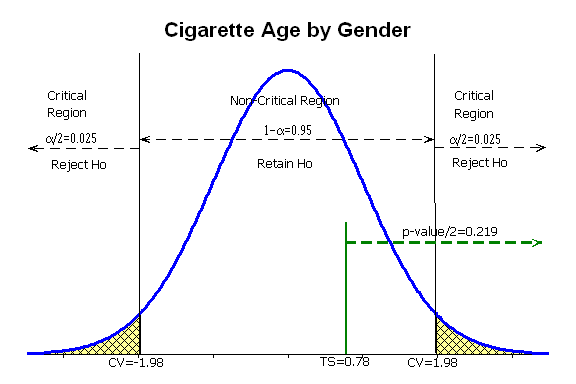# Age at which People Start Smoking

This is not a complete project. Many students often struggle with the hypothesis testing portion of the project, but this is the major reason for doing the project. The following is an example of how to structure that section.

## Sample Inferential Statistics

The claim that we're testing is that men and women try cigarettes at the same age. Written symbolically, this is μf = μm and is the null hypothesis. Therefore, the null and alternative hypotheses are:

H0: μf = μm
H1: μf ≠ μm

Minitab was used to conduct a t-test for two independent samples.

### Two-sample T for cigarette

```gender   N   Mean  StDev  SE Mean
Female  78  15.14   2.80     0.32
Male    72  14.76   3.11     0.37
```
```Difference = mu (Female) - mu (Male)
Estimate for difference:  0.377
95% CI for difference:  (-0.581, 1.335)
T-Test of difference = 0 (vs not =): T-Value = 0.78  P-Value = 0.438  DF = 143```

We have a two tail test and there are 143 df, so the the critical values for an α = 0.05 signficance level are t = ±1.976692.The test statistic of t = 0.78 falls in the non-critical region, so we retain the null hypothesis. The pvalue of 0.438 is greater than the significance level of 0.05, so we retain the null hypothesis. The claimed difference of 0 falls in the confidence interval of -0.581 < μf - μm < 1.335 so we retain the null hypothesis. In all three approaches to hypothesis testing, we retain the null hypothesis.

There is not enough evidence to reject the claim that the age at which men and women first try cigarettes is the same. There is not enough evidence to support the claim that the age at which men and women first try cigarettes is different.